• 遗传算法python库Genetic algorithms (GA) are an optimization and search technique based on the principles of genetics and natural selection, in essence mimicking the natural evolution process that we ...
遗传算法python库Genetic algorithms (GA) are an optimization and search technique based on the principles of genetics and natural selection, in essence mimicking the natural evolution process that we observe in life. Their general principle is based on the concept of having an initial population composed of several individuals — with each representing a particular solution to the problem — and allow it to evolve to a state that maximizes its overall fitness, using three main operators: selection, crossover and mutation. We’ll look into these aspects a bit more in detail below. 遗传算法(GA)是一种基于遗传和自然选择原理的优化和搜索技术，实质上模仿了我们在生活中观察到的自然进化过程。 他们的一般原则基于以下概念： 初始人口由几个人组成，每个人代表一个特定的问题解决方案，并使用三个主要运算符将其发展为最大化整体适应性的状态： 选择 ， 交叉和突变 。 我们将在下面更加详细地研究这些方面。
Genetic Algorithms are nothing short of fantastic, as they can be applied to many kinds of optimization problems and find solutions to complex functions for which we do not have a mathematical expression. This comes at a cost of computational complexity though, as, for large populations, we’ll have to evaluate the fitness of all individuals at every generation. If the fitness function is expensive, the algorithm run will be slow. 遗传算法简直就是太神奇了，因为它们可以应用于多种优化问题并找到我们没有数学表达式的复杂函数的解。 但是，这是以计算复杂性为代价的，因为对于大量人口，我们必须评估每一代所有个体的适应性。 如果适应度函数昂贵，则算法运行会很慢。
GA’s can be divided into Binary and Continuous, depending on the type of problem we’re optimizing for. Potentially all problems could be broken down as having their variables (genes) represented by binary strings, but in general, if the input space is real-valued, it makes more sense to use a continuous GA. GA可以分为Binary和Continuous ，具体取决于我们要优化的问题类型。 可能所有问题都可以通过用二进制字符串表示其变量( 基因)来分解，但是总的来说，如果输入空间是实值，则使用连续 GA更有意义。
As there are fewer examples for continuous GA out there, the examples shown here will be for that version of GA. 由于那里连续GA的示例较少，因此此处显示的示例适用于该版本的GA。
Initialization 初始化
The search starts with a random population of N individuals. Each of those individuals corresponds to a chromosome, which encodes a sequence of genes representing a particular solution to the problem we’re trying to optimize for. Depending on the problem at hand, the genes representing the solution could be bits (0’s and 1’s) or continuous (real valued). An example of a real-valued chromosome representing a solution to a given problem with 9 variables (genes) is shown below. 搜索从N个个体的随机种群开始。 这些个体中的每一个都对应一条染色体 ，该染色体编码表示代表我们要优化的问题的特定解决方案的一系列基因。 根据当前的问题，代表解决方案的基因可以是位(0和1)或连续(实值)。 下面显示了一个实值染色体的示例，该染色体代表具有9个变量( 基因 )的给定问题的解决方案。Example of an individual’s chromosomeFitness 适合度
The fitness of each individual is what defines what we are optimizing for, so that, given a chromosome encoding a specific solution to a problem, its fitness will correspond to how well that particular individual fares as a solution to the problem. Therefore, the higher its fitness value, the more optimal that solution is. 每个人的健康状况   是什么定义了我们要优化的内容，因此，给定染色体编码的问题的特定解决方案，其适应性将对应于特定个体解决问题的能力。 因此，其适用性值越高，该解决方案越理想。
After all, individuals have their fitness score calculated, they are sorted, so that the fittest individuals can be selected for crossover. 毕竟，已经计算了个人的健身得分，并对他们进行了排序，以便可以选择最适合的个人进行交叉。
Selection 选拔
Selection is the process by which a certain proportion of individuals are selected for mating between each other and create new offsprings. Just like in real-life natural selection, individuals that are fitter have higher chances of surviving, and therefore, of passing on their genes to the next generation. Though versions with more individuals exist, usually the selection process matches two individuals, creating pairs of individuals. There are four main strategies: 选择是选择特定比例的个体以使其彼此交配并产生新后代的过程。 就像现实中的自然选择一样，更健康的个体有更高的生存机会，因此可以将其基因传给下一代。 尽管存在更多个人的版本，但通常选择过程会匹配两个个人，从而创建成对的个人。 有四种主要策略：
pairing: This is perhaps the most straightforward strategy, as it simply consists of pairing the top fittest chromosomes two-by-two (pairing odd rows with even ones). 配对 ：这可能是最直接的策略，因为它仅包括将最合适的染色体两两配对(将奇数行与偶数行配对)。
random: This strategy consists of randomly selecting individuals from the mating pool. 随机 ：此策略包括从交配池中随机选择个体。
roulette wheel: This strategy also follows a random principle, but fitter individuals have higher probabilities of being selected. 轮盘赌 ：这种策略也遵循随机原则，但是更健康的人被选中的可能性更高。
tournament: With this strategy, the algorithm first selects a few individuals as candidates (usually 3), and then selects the fittest individual. This option has the advantage that it does not require the individuals to be sorted by fitness first. 比赛 ：采用这种策略时，算法首先选择一些个人作为候选者(通常为3个人)，然后选择最适合的个人。 该选项的优点是它不需要先按适合度对个人进行分类。
A python implementation for the roulette wheel strategy is shown on the snippet below. 下面的代码段显示了轮盘策略的python实现。
Crossover 交叉
This is the step where new offsprings are generated, which will then replace the least fit individuals in the population. The idea behind crossing over individuals is that, by combining different genes, we might produce even fitter individuals, which will be better solutions to our problem. Or not, and in that case, those solutions won’t survive to the next generations. 这是生成新后代的步骤，然后将替换种群中最不适合的个体。 跨越个体​​背后的想法是，通过组合不同的基因，我们甚至可以生产出更健康的个体，这将是解决我们问题的更好方法。 不管是不是，在那种情况下，这些解决方案都无法延续到下一代。
In order to perform the actual crossover, each of the pairs coming from the selection step are combined to produce two new individuals each, which will both have genetic material from each of the parents. There are several different strategies for performing the crossover, so for brevity, we’ll only discuss one of them. 为了进行实际的杂交，将来自选择步骤的每对配对以产生两个新个体，每个个体都具有来自每个亲本的遗传物质。 执行交叉有几种不同的策略，为简便起见，我们仅讨论其中一种。
Supposing we have a problem defined by 9 variables, if we have 2 parents and we choose randomly the crossover gene as index 3, then each of the offsprings will be a combination of each parent, as shown in the diagram below. 假设我们有一个由9个变量定义的问题，如果我们有2个父母，并且我们随机选择交叉基因作为索引3，那么每个后代将是每个父母的组合，如下图所示。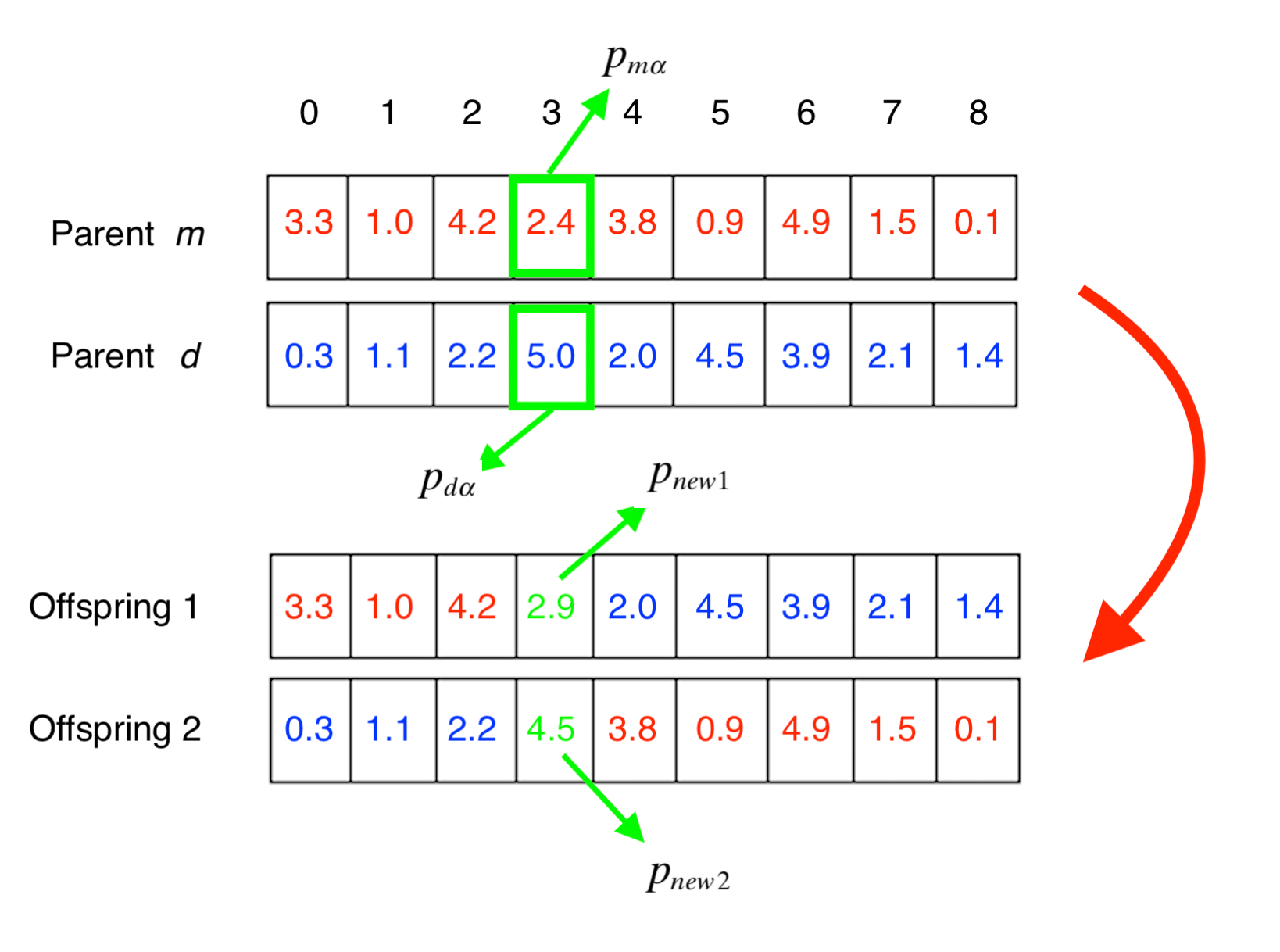Diagram showing how parents are crossed over to generate new offspringThe crossover gene of each offspring is calculated according to the rule given by: 每个后代的交叉基因根据以下规则计算：Equation for calculating new crossover genesWhere β will be a random number between 0 and 1. The python code for the crossover is given below. 其中β是0到1之间的随机数。下面给出了交叉的python代码。
Mutation 突变
Mutation is the process by which we introduce new genetic material in the population, allowing the algorithm to search a larger space. If it were not for mutation, the existing genetic material diversity in a population would not increase, and, due to some individuals “dying” between generations, would actually be reduced, with individuals tending to become very similar quite fast. 突变是我们在种群中引入新遗传物质的过程，从而使算法可以搜索更大的空间。 如果不是为了突变，则种群中现有的遗传物质多样性将不会增加，而且由于某些个体在世代之间“垂死”，实际上会减少，而个体趋于变得非常相似。
In terms of the optimization problem, this means that without new genetic material the algorithm can converge to local optima before it explores an enough large size of the input space to make sure that we can reach the global optimum. Therefore, mutation plays a big role in maintaining diversity in the population and allowing it to evolve to fitter solutions to the problem. 就优化问题而言，这意味着在没有新的遗传材料的情况下，该算法可以先收敛到局部最优，然后再探索足够大的输入空间，以确保我们可以达到全局最优。 因此，突变在维持种群多样性并使其进化以适应问题的解决方案中起着重要作用。
The most simple way we can do this is, given a certain mutation rate, to randomly choose some individuals and some genes and assign a new random number to those positions. This is exemplified in the diagram and code snippet below. 我们可以执行此操作的最简单方法是，在确定突变率的情况下 ，随机选择一些个体和某些基因，并为这些位置分配一个新的随机数。 下面的图和代码段中对此进行了举例说明。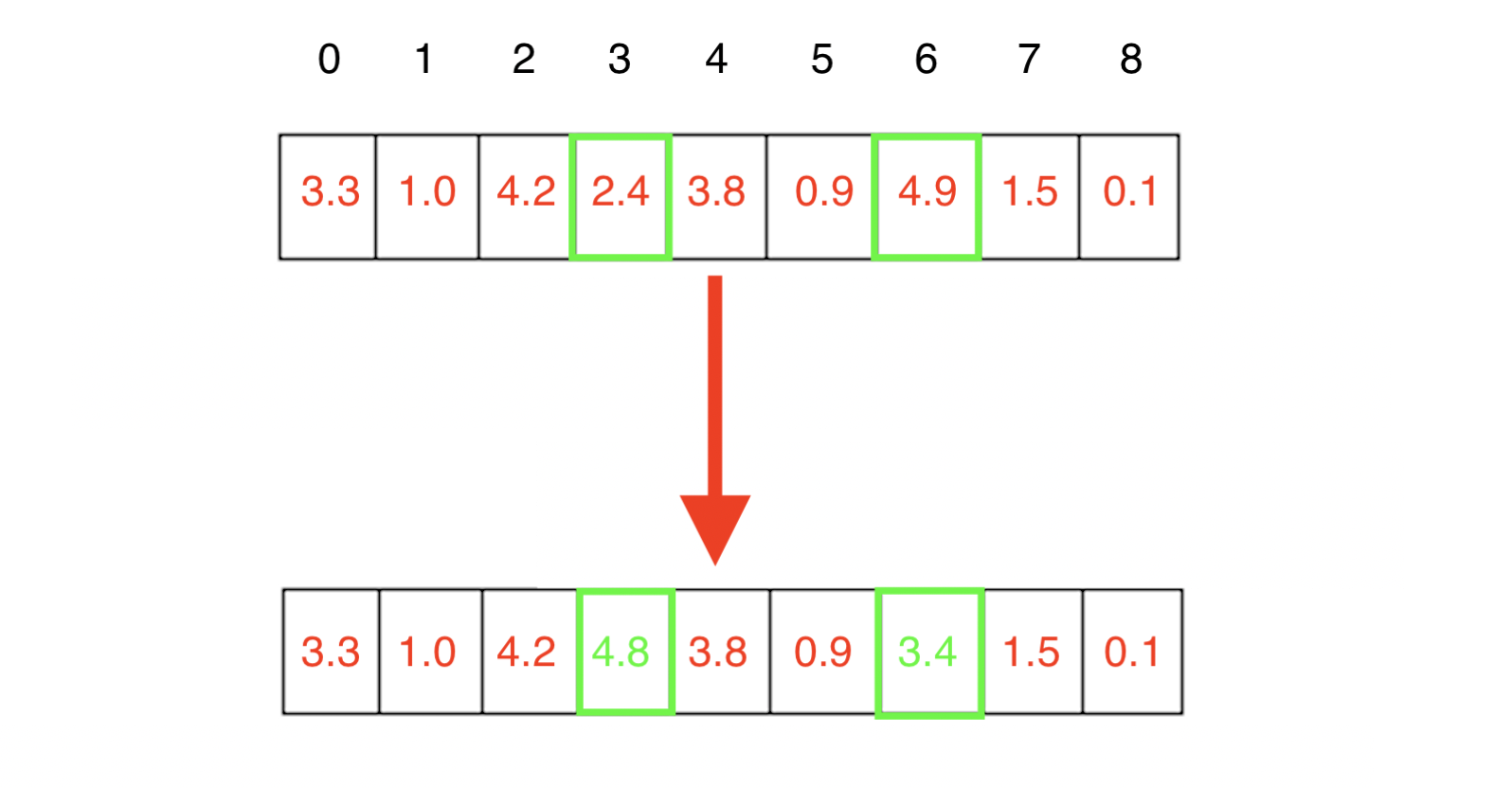Mutation of two genes in an individualSolver 解算器
Now it’s time to tie it all together. Using the operators that we defined above, the algorithm can now solve the problem, with the actual main cycle of the algorithm being implemented in just a few lines of code. The flowchart of the algorithm, as well as an example implementation in python are shown below. 现在是时候将它们捆绑在一起了。 使用上面定义的运算符，该算法现在可以解决问题，该算法的实际主循环仅用几行代码即可实现。 该算法的流程图以及python中的示例实现如下所示。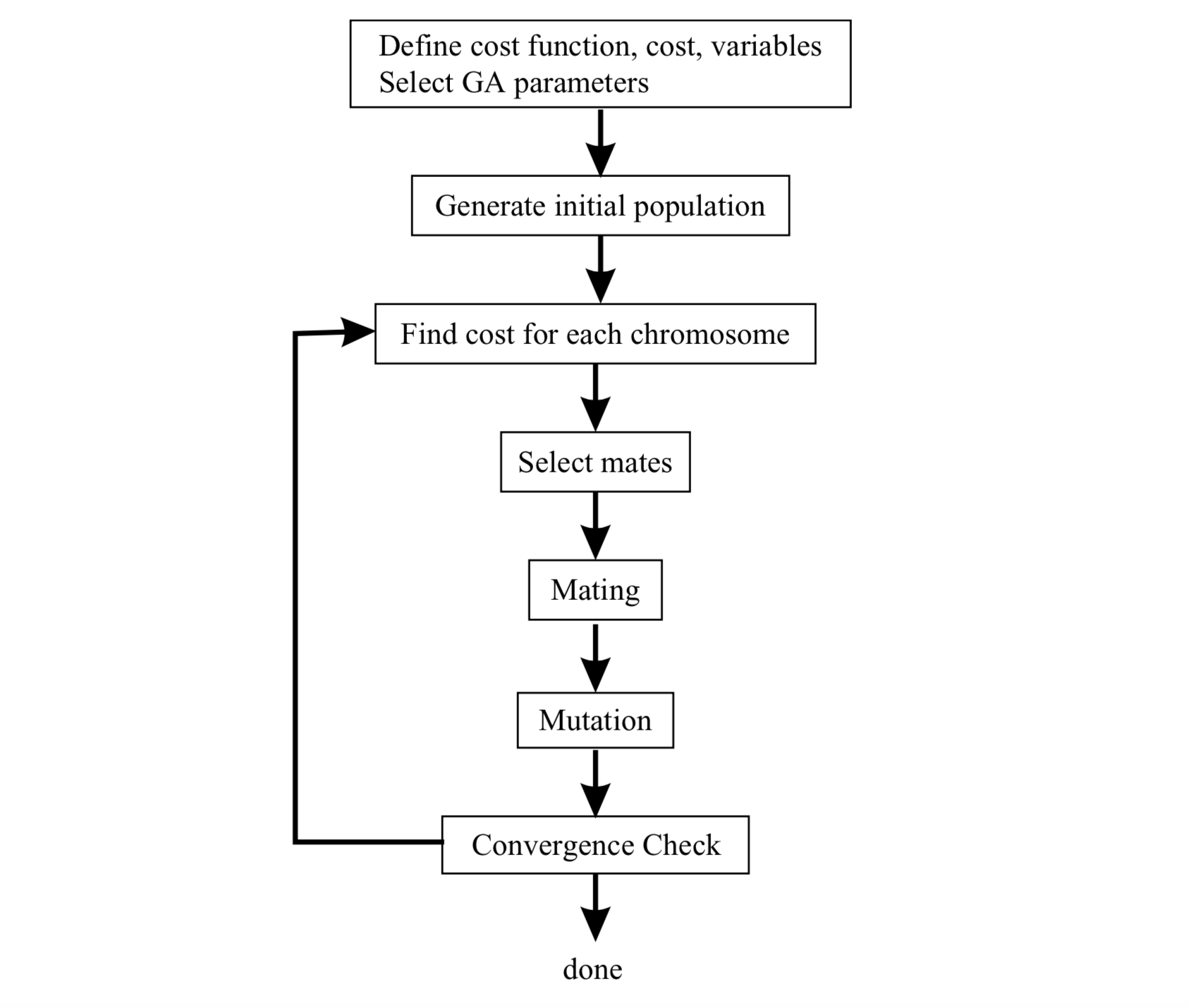Flowchart of the Genetic Algorithm 介绍GeneAl (Introducing GeneAl)
GeneAl is a python library implementing Genetic Algorithms, which can be used and adapted to solve many optimization problems. One can use the provided out-of-the-box solver classes — BinaryGenAlgSolver and ContinuousGenAlgSolver — , or create a custom class which inherits from one of these, and implements methods that override the built-in ones. It also has support for solving the (in)famous Travelling Salesman Problem. GeneAl是实现遗传算法的python库，可用于解决许多优化问题。 可以使用提供的现成的求解器类BinaryGenAlgSolver和ContinuousGenAlgSolver ，或者创建一个自定义类继承自其中的类，并实现覆盖内置方法的方法。 它还支持解决著名的旅行推销员问题 。
For brevity, we’ll only see how to use the continuous version — keeping in line with this post — , but for more details, check out the README of this project. 为简便起见，我们只会看到如何使用连续版本-与本文保持一致-，但是要了解更多详细信息，请查看该项目的自述文件。
The first thing would be to install the package, which can be made through pip, as such: 首先是要安装软件包，可以通过pip进行安装，如下所示：
pip install geneal After the installation completes, one is ready to use it. So let’s see how we can use the ContinuousGenAlgSolver class. 安装完成后，就可以使用了。 因此，让我们看看如何使用ContinuousGenAlgSolver类。
As a bare minimum, the class requires the user to provide the number of genes present in the problem, as well as to provide the custom defined fitness function. For convenience, the library provides some default fitness functions that can be used for testing. 至少，该类别要求用户提供问题中存在的基因数量，并提供自定义的适应度函数。 为了方便起见，该库提供了一些可用于测试的默认适应性函数。
With the initialization done above, the class will solve the problem using default values for all the parameters. If we wish to have more control over the algorithm run, we will want to adjust these, and that can be done as shown below: 通过上面的初始化，该类将使用所有参数的默认值解决问题。 如果我们希望对算法的运行有更多的控制，我们将希望对其进行调整，如下所示：
Finally, this class allows the user to specify the type of problem — if the possible values are integers or floats — , as well as the variables’ limits, in order to limit the search space. 最后，该类允许用户指定问题的类型(如果可能的值是整数或浮点数)以及变量的限制，以限制搜索空间。
This completes this short introduction to the library. If you want to know more, check out the GitHub repository, which has more information :) 这样就完成了对库的简短介绍。 如果您想了解更多信息，请查看GitHub存储库 ，其中包含更多信息:)
Thanks for reading! 谢谢阅读！
翻译自: https://towardsdatascience.com/introducing-geneal-a-genetic-algorithm-python-library-db69abfc212c遗传算法python库
展开全文人工智能
• ## 遗传算法Python实现

千次阅读 2019-05-25 17:23:13
遗传算法Python实现遗传算法Python实现导入库以及参数设置目标函数生成C行R列的值在0-1的数组混沌函数二进制转十进制个体按值从大到小排序交叉变异适应度函数主函数 遗传算法Python实现 导入库以及参数设置 import ...
遗传算法Python实现瞎BB代码导入库以及参数设置目标函数生成C行R列的值在0-1的数组混沌函数二进制转十进制个体按值从大到小排序交叉变异适应度函数主函数
瞎BB
代码
导入库以及参数设置
import pandas as pd
import numpy as np
import matplotlib.pyplot as plt
import math
import random

#range of variable
bounds = np.array([-2,2])
#begin of variable
boundsbegin = bounds
#end of variable
boundsend = bounds
precision = 0.0001
#calc the BitLength
BitLength = math.ceil(np.log2((boundsend - boundsbegin) / precision))
#init
popsize = 100
Generationmax = 100
pmut = 0.09

目标函数
def targetfun(x):
value = 200 * math.exp(-0.05 * x) * math.sin(x)
return value

生成C行R列的值在0-1的数组
def random_random(C,R):
rand=[]
for i in range(C*R):
rand.append(random.random())
rand=np.array(rand)
return rand.reshape(C,R)

混沌函数
def chaos(size):
chaos_cro = np.zeros(size)
chaos_cro = random.random()
for j in range(1,size):
chaos_cro[j] = 4 * chaos_cro[j - 1] * (1 - chaos_cro[j - 1])
return chaos_cro[-1]

二进制转十进制
def transform2to10(sample):
BitLength = len(sample)
x=sample[-1]
for i in range(1,BitLength):
x=x+sample[-i-1]*np.power(2,i)
return x

个体按值从大到小排序
def rank(population):
popsize = population.shape
BitLength = population.shape
fitvalue = np.zeros(popsize).reshape(popsize,1)
for i in range(popsize):
x = transform2to10(population[i])
#tansform to range of variable
xx=boundsbegin + x * (boundsend - boundsbegin) / (np.power(boundsend,BitLength)-1)
fitvalue[i,0]=targetfun(xx)
#make fitvalue(j)<fitvalue(j+1)
res = np.concatenate((population,fitvalue),axis = 1)
res=pd.DataFrame(res)
population = res.sort_values(by=BitLength)
population = np.array(population)
population = population[:,0:BitLength]
return population

交叉变异
def cro_mut_improve(population,rate_mut):
#seln:two individuals
popsize = population.shape
BitLength = population.shape
population = rank(population)
#crossover
pop=popsize
chaos_cro = np.zeros(BitLength)

if popsize % 2 == 1:
pop = popsize - 1

for i in range(0,pop,2):
chaos_cro = chaos(BitLength)
chao = math.floor(chaos_cro * BitLength)
temp = population[i,chao]
population[i,chao] = population[i+1,chao]
population[i + 1,chao] = temp

#mutation
by = np.array([])
n = len(by)
#generate random individuals to mutate
while n == 0:
by = random_random(1,popsize)<rate_mut
for i in by:
if i:
n+=1
num_mut = n
for k in range(popsize):
if by[0,k] == True:
chaos_mut = np.zeros(BitLength)
chaos_mut = random.random()
for t in range(1,BitLength):
chaos_mut[t] = 4 * chaos_mut[t - 1] * (1 - chaos_mut[t - 1])
position = np.floor(BitLength * random_random(1,2))
position.astype(np.int16)
population[k,int(position[0,0])] = np.round(chaos(BitLength))
population[k,int(position[0,1])] = np.round(chaos(BitLength))
return population

适应度函数
def fitness(population):
#population=pd.DataFrame(population)
popsize = population.shape
BitLength = population.shape
cumsump = np.zeros(popsize).reshape(1,popsize)
fitvalue = np.zeros(popsize).reshape(1,popsize)
for i in range(popsize):
x = transform2to10(population[i])
#tansform to range of variable
xx = boundsbegin + x * (boundsend - boundsbegin) / (np.power(boundsend,BitLength) - 1)
fitvalue[0,i] = targetfun(xx)
#ensure fitvalue>0
fitvalue = fitvalue+230
fsum = fitvalue.sum(1)
Pperpopulation = fitvalue / fsum
cumsump = Pperpopulation
for i in range(1,popsize):
cumsump[0,i] = cumsump[0,i-1] + Pperpopulation[0,i]
res = np.concatenate((fitvalue,cumsump),axis = 0)
return res

主函数
ymax=np.zeros(Generationmax+1)
ymean=np.zeros(Generationmax+1)
xmax=np.zeros(Generationmax+1)
#generate random population
population = np.round(random_random(popsize,BitLength))
#calc fitness return fitvalue and sum of probability
res=fitness(population)
fitvalue = res
cumsump = res
#main code
Generation=1
while Generation<Generationmax+1:
population=cro_mut_improve(population,pmut)
res=fitness(population)
fitvalue = res
cumsump = res
fmax = np.max(fitvalue)
for i in range(popsize):
if fitvalue[i]==fmax:
nmax = i
break
fmean = np.mean(fitvalue,axis = 0)
ymax[Generation] = fmax
ymean[Generation] = fmean
x = transform2to10(population[nmax])
xx = boundsbegin + x * (boundsend-boundsbegin) / (pow(boundsend,BitLength)-1)
xmax[Generation] = xx
Generation += 1
#Generation=Generation-1;
Bestpopulation = np.max(xmax)
BestValue = targetfun(Bestpopulation)
print(Bestpopulation)
print(BestValue)

x=[]
y=[]
for i in range(400):
t=-2+0.01*i
x.append(t)
y.append(targetfun(t))
plt.scatter(x, y)



展开全文优化
• 遗传算法python实现 遗传算法（也称为“ GA”）是受查尔斯·达尔文（Charles Darwin）的自然选择理论启发而提出的算法，旨在为我们不太了解的问题找到最佳解决方案。 例如：当您不能推导给定函数的最大值或最小值时...

遗传算法python实现 遗传算法（也称为“ GA”）是受查尔斯·达尔文（Charles Darwin）的自然选择理论启发而提出的算法，旨在为我们不太了解的问题找到最佳解决方案。 例如：当您不能推导给定函数的最大值或最小值时，如何找到它？ 它基于三个概念： 选择 ， 复制和变异 。 我们随机生成一个个体集合， 选择最好的， 在最后越过它们稍微变异的结果-一遍又一遍，直到我们找到一个可接受的解决方案。 您可以在Goldberg的书中检查其他搜索方法的一些比较。
让我们检查一下如何使用Python编写遗传算法的简单实现！

我们将在这里尝试解决的问题是找到类似于帽子的3D功能的最大值。 它定义为f（x，y）= sin（sqrt（x ^ 2 + y ^ 2）） 。 我们将问题限制在4≥x≥- 4和4≥y≥- 4的边界上。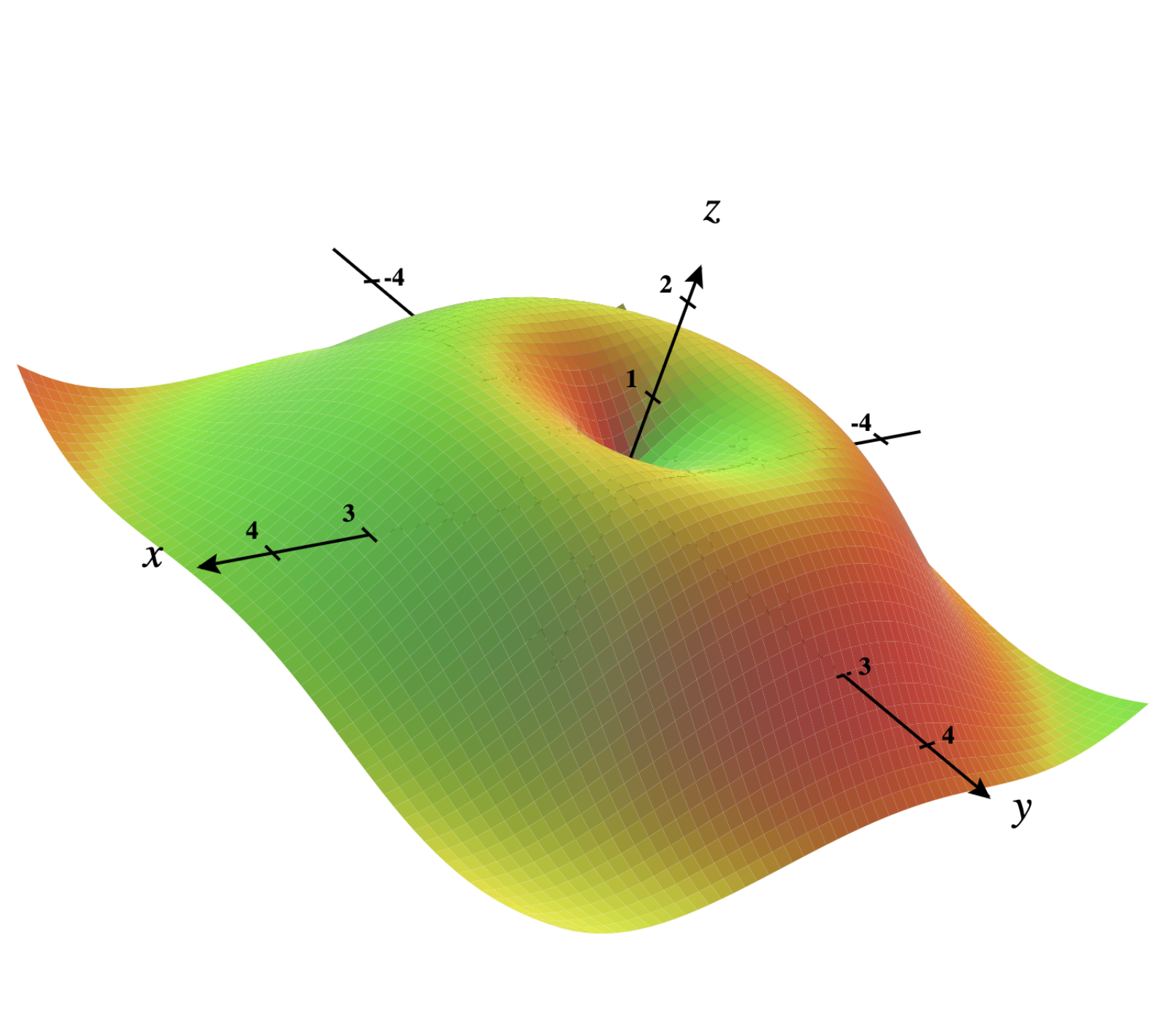（ 函数定义在我们定义的边界之间，由 CalcPlot3D 创建 ）
第一步是生成我们的初始人口。 人口或世代是我们当前称为个人的可能解决方案集。 我们将反复进行几代的改进，直到找到可接受的解决方案。 第一代是随机生成的。
import random

def generate_population (size, x_boundaries, y_boundaries) :
lower_x_boundary, upper_x_boundary = x_boundaries
lower_y_boundary, upper_y_boundary = y_boundaries

population = []
for i in range(size):
individual = {
"x" : random.uniform(lower_x_boundary, upper_x_boundary),
"y" : random.uniform(lower_y_boundary, upper_y_boundary),
}
population.append(individual)

return population
我们的创始函数需要三个参数：人口应该拥有的个体数量，一个元组指示x轴上的边界，一个元组指示y轴上的边界，因此我们的个体随机地拟合这些边界。
继续，让我们定义健身功能。 这将是我们的评估者，它将表达一个人彼此之间的好坏程度。 身体最好的人应该得到保护和繁殖，而最坏的人应该跌倒-就像自然界一样。 在我们的案例中，我们要如何找到函数的最大值，我们可以简单地将目标函数应用于个人，最大的数字也将是最大的适用性。 如果我们想找到最小值，则适应度可以表示为功能时间-1的结果，因此较小的值将变为较大的适应度。
import math

def apply_function (individual) :
x = individual[ "x" ]
y = individual[ "y" ]
return math.sin(math.sqrt(x ** 2 + y ** 2 ))
由于我们拥有人口产生器和健康评估器，因此我们可以开始繁殖我们的个体以实现下一代。 我们将一直这样做，直到找到可接受的解决方案。 停止条件有好几种，其中一个被广泛使用的标准是“ n代具有陈旧的适应性”，但我们将使用一个更简单的标准，即n代-我们将使用100。到目前为止，我们的输入函数如下：
generations =100

population = generate_population(size= 10 , x_boundaries=( -4 , 4 ), y_boundaries=( -4 , 4 ))

i = 1
while True :
print( f"🧬 GENERATION {i} " )

for individual in population:
print(individual)

if i == generations:
break

i += 1

# Make next generation...
为了选择要复制的个体，我们将使用一种被广泛采用的方法，称为轮盘赌法，该方法包括将圆分成多个部分，例如饼图，其中每个个体的比例都与其适应度成正比，然后旋转它。 通过这种方式，我们可以确保最好的个人有更好的机会被选中，而最坏的人仍然有机会，尽管这是次要的。
def choice_by_roulette (sorted_population, fitness_sum) :
offset = 0
normalized_fitness_sum = fitness_sum

lowest_fitness = apply_function(sorted_population[ 0 ])
if lowest_fitness < 0 :
offset = -lowest_fitness
normalized_fitness_sum += offset * len(sorted_population)

draw = random.uniform( 0 , 1 )

accumulated = 0
for individual in sorted_population:
fitness = apply_function(individual) + offset
probability = fitness / normalized_fitness_sum
accumulated += probability

if draw <= accumulated:
return individual
为了说明我们的方法，假设我们有四个个人：A，B，C和D分别具有0、50、200和250的适应度。 总适应度的总和为500，因此每个人都有被选择适应度/ total_fitness的机会：0％，10％，40％，50％。 我们选择一个介于0和1之间的随机数，然后验证哪个人在所选部分中：A [0，0]，B（0，0.1]，C（0.1，0.5]，D（0.5，1]。
由于我们的方案可能具有负适应性，因此我们首先必须通过选择最低适应性，乘以-1并将其相加来对我们的个体进行归一化（例如，如果我们有两个个体的适应性分别为-10和5，我们将10加为0和15）。 我们还希望将人口论证按照适合度升序排列，因此更容易找到最坏和最好的人。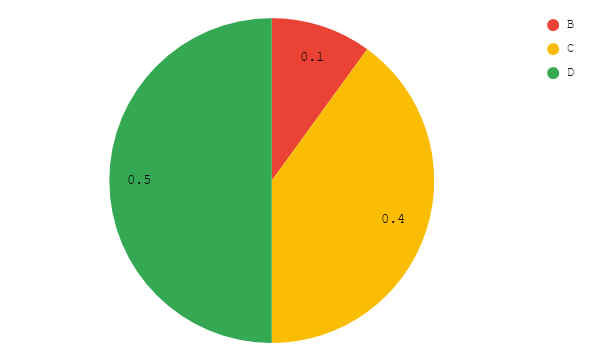然后让我们填充下一代。 它的长度应与第一个相同，因此我们将重复10次，使用轮盘赌选择两个人，然后越过他们。 结果产生的个体将受到较小的扰动（变异），因此我们不会坚持舒适区，而是要寻找比目前为止更好的解决方案。
实数有几种交叉技术：例如，我们可以取个体A的x和个体B的y ，可以取每个的几何平均值，或者最简单的取每个的算术平均值。 如果要处理二进制数据，最常用的技术是选择A的一部分位串和B的一部分位。出于简单起见，让我们使用算术平均值。
对于突变也有很多选择-我们将简单地将固定间隔之间的一个小的随机数求和。 该间隔是突变率，可以相应地进行微调，让我们使用[-0.05，0.05]。 对于较大的搜索空间，您可以选择较大的间隔并逐代减小。 处理二进制数据时，您可以简单地翻转单个字符串的随机选择的位。
def sort_population_by_fitness (population) :
return sorted(population, key=apply_function)

def crossover (individual_a, individual_b) :
xa = individual_a[ "x" ]
ya = individual_a[ "y" ]

xb = individual_b[ "x" ]
yb = individual_b[ "y" ]

return { "x" : (xa + xb) / 2 , "y" : (ya + yb) / 2 }

def mutate (individual) :
next_x = individual[ "x" ] + random.uniform( -0.05 , 0.05 )
next_y = individual[ "y" ] + random.uniform( -0.05 , 0.05 )

lower_boundary, upper_boundary = ( -4 , 4 )

# Guarantee we keep inside boundaries
next_x = min(max(next_x, lower_boundary), upper_boundary)
next_y = min(max(next_y, lower_boundary), upper_boundary)

return { "x" : next_x, "y" : next_y}

def make_next_generation (previous_population) :
next_generation = []
sorted_by_fitness_population = sort_population_by_fitness(previous_population)
population_size = len(previous_population)
fitness_sum = sum(apply_function(individual) for individual in population)

for i in range(population_size):
first_choice = choice_by_roulette(sorted_by_fitness_population, fitness_sum)
second_choice = choice_by_roulette(sorted_by_fitness_population, fitness_sum)

individual = crossover(first_choice, second_choice)
individual = mutate(individual)
next_generation.append(individual)

return next_generation
就是这样！ 现在，我们有了GA的所有三个步骤：选择，交叉和突变。 我们的主要方法就是这样：
generations =100

population = generate_population(size= 10 , x_boundaries=( -4 , 4 ), y_boundaries=( -4 , 4 ))

i = 1
while True :
print( f"🧬 GENERATION {i} " )

for individual in population:
print(individual, apply_function(individual))

if i == generations:
break

i += 1

population = make_next_generation(population)

best_individual = sort_population_by_fitness(population)[ -1 ]
print( "\n🔬 FINAL RESULT" )
print(best_individual, apply_function(best_individual))
在这100代之后， best_individual变量将使我们的个体适应度最高。 不管它是否是精确的最佳解决方案，您都必须微调参数（变异率，世代等）和技术（选择，交叉和变异方法），直到无法改善为止。 让我们看一下实验运行的最后输出行（请注意，由于随机参数，您很可能会获得不同但相似的结果）：
🧬 GENERATION 100
{'x': -1.0665224807251312, 'y': -1.445963268888755} 0.9745828000809058
{'x': -1.0753606354537244, 'y': -1.4293367491155182} 0.976355423070003
{'x': -1.0580786664161246, 'y': -1.3693549033564183} 0.9872729309456848
{'x': -1.093601208942564, 'y': -1.383292089777704} 0.9815156357267611
{'x': -1.0464963866796362, 'y': -1.3461172606906064} 0.9910018621648693
{'x': -0.987226479369966, 'y': -1.4569537217049857} 0.9821687265560713
{'x': -1.0501568673329658, 'y': -1.430577408679398} 0.9792937786319258
{'x': -1.0291192465186982, 'y': -1.4289167102720242} 0.9819781801342095
{'x': -1.098502968808768, 'y': -1.3738230550364259} 0.9823409690311633
{'x': -1.091317403073779, 'y': -1.4256574643591997} 0.9748817266026281

🔬 FINAL RESULT
{'x': -1.0464963866796362, 'y': -1.3461172606906064} 0.9910018621648693
我们的最终结果非常接近一种可能的解决方案（此函数在我们的边界内具有多个最大值，如开始时在图上所见，该最大值为1.0）。 请注意，我们使用的是不太复杂的技术，因此可以预期得到此结果-这是微调的起点，直到我们能够找到世代较少的更好的解决方案为止。

这是一篇有关使用Python的遗传算法的入门文章。 如果您喜欢它，您肯定会想更多地了解您可以对其进行的所有可能的改进以及可以使用它的应用程序。 我强烈建议阅读开头提到的“ 搜索，优化和机器学习中的遗传算法 ”这本书。
翻译自: https://hackernoon.com/genetic-algorithms-explained-a-python-implementation-sd4w374i遗传算法python实现

展开全文人工智能 机器学习 java
• 遗传算法 粒子群算法 模拟退火算法 蚁群算法 免疫优化算法 人工鱼群算法 项目链接： https://github.com/zhangxiao123qqq/scikit-opt 原作者链接： https://github.com/guofei9987/scikit-opt 黑盒实现，即拿即用！...
工具包
在GitHub上找到一个开源的项目，并对其进行了更新，提交了一些小bug，它实现了数学建模中常用的算法包括：

差分进化算法
遗传算法
粒子群算法
模拟退火算法
蚁群算法
免疫优化算法
人工鱼群算法

项目链接：
https://github.com/zhangxiao123qqq/scikit-opt
原作者链接：
https://github.com/guofei9987/scikit-opt
黑盒实现，即拿即用！不要太爽！！
提示：项目中使用等help()命令可直接获得参数说明。
#遗传算法帮助
help(GA)
#模拟退火算法帮助
help(SA)

算法使用方式见：
中文使用文档
Demo演示:
demo_ga
import numpy as np

def schaffer(p):
'''
This function has plenty of local minimum, with strong shocks
global minimum at (0,0) with value 0
'''
x1, x2 = p
x = np.square(x1) + np.square(x2)
return 0.5 + (np.square(np.sin(x)) - 0.5) / np.square(1 + 0.001 * x)

# %%
from sko.GA import GA

ga = GA(func=schaffer, n_dim=2, size_pop=50, max_iter=800, lb=[-1, -1], ub=[1, 1], precision=1e-7)
best_x, best_y = ga.run()
print('best_x:', best_x, '\n', 'best_y:', best_y)

# %% Plot the result
import pandas as pd
import matplotlib.pyplot as plt

Y_history = pd.DataFrame(ga.all_history_Y)
fig, ax = plt.subplots(2, 1)
ax.plot(Y_history.index, Y_history.values, '.', color='red')
Y_history.min(axis=1).cummin().plot(kind='line')
plt.show()



展开全文数学建模
• 遗传算法python实现一、问题引入二、遗传算法的步骤1.初始化2.个体评价3.选择运算4.交叉运算5.变异运算6.终止条件判断三、实现思路1.编码的设计2.适应度函数3.选择函数4.交叉函数5.变异函数6.迭代四、具体实现1.编码...算法
• 本文实例为大家分享了python遗传算法的具体代码，供大家参考，具体内容如下 1、基本概念 遗传算法（GA）是最早由美国Holland教授提出的一种基于自然界的“适者生存，优胜劣汰”基本法则的智能搜索算法。该法则很好...
• 遗传算法Python程序 Hands-On-Genetic-Algorithms-with-Python-master.zip
• 遗传算法Python 教程（1） 遗传算法简介 遗传算法是一种通过种群演化得到最优解的搜索算法。遗传算法受启发与生物演化过程中的一些现象，这些现象包括自然选择，交配，突变，遗传等。本教程将通过Python来展示遗传...教程
• 遗遗传传算算法法python版版 这篇文章主要为大家详细介绍了python实现遗传算法具有一定的参考价值感兴趣的小伙伴们可以参考一下 本文实例为大家分 了python遗传算法的具体代码供大家参考具体内容如下 1基基本本概...
• Python实现这些算法的集合：差分进化算法、遗传算法、粒子群算法、模拟退火算法、蚁群算法、免疫优化算法、鱼群算法
• 科学计算之遗传算法python实现☞1遗传算法染色体编码和种群初始化适应度和选择选择交配选择变异代码主体目标函数运行效果 版权声明：本文为威哥哥带你写代码原创文章，有错请评论，转载请注明，谢谢 遗传算法 遗传...
• 遗传算法Python例程，以寻找最大值为例，另附学习笔记更利于学习和记忆
• 解决的例子y=10*sin(5x)+7*cos(4x) 代码用python3.6可以运行
• ## 遗传算法Python实现

千次阅读 多人点赞 2020-07-08 15:23:46
> 本文中的遗传算法是之前所写，为10个种群，10个染色体的遗传算法实现。 > 适应值函数为di的累加 > 其余各项在代码中有注释，有需要的话可以自行查看，仅为遗传算法实现的一个示例。
• 遗传算法Python实现一、GA算法介绍二、GA算法流程三、Python实现四、优缺点及应用1、不足之处2、特点3、应用 一、GA算法介绍   遗传算法（Genetic Algorithm，GA）最早是由美国的 John holland于20世纪70年代提出,...
• 遗传算法python代码（附详细注释） #代码参考：https://blog.csdn.net/ha_ha_ha233/article/details/91364937 import numpy as np #用于数据操作：【X = np.linspace(*X_BOUND, 100) #将列表传入收集参数，完成解包...
• 柔性作业车间调度问题中，以完工时间作为优化目标，编写的遗传算法代码，python语言。代码中套用了一个自己随机生成的实例进行运行验证，仅供参考学习。...

# 遗传算法pythonpython 订阅